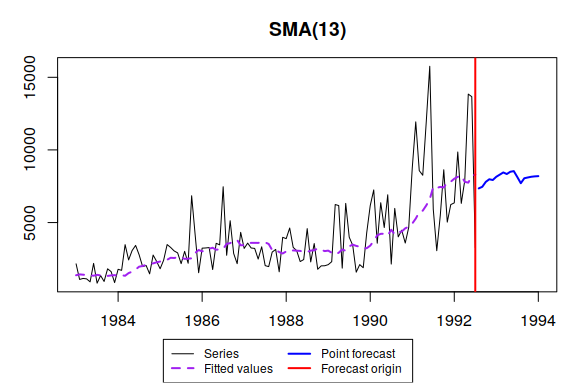# sma() - Simple Moving Average

#### 2019-10-22

Simple Moving Average is a method of time series smoothing and is actually a very basic forecasting technique. It does not need estimation of parameters, but rather is based on order selection. It is a part of smooth package.

In this vignette we will use data from Mcomp package, so it is advised to install it.

require(smooth)
require(Mcomp)

You may note that Mcomp depends on forecast package and if you load both forecast and smooth, then you will have a message that forecast() function is masked from the environment. There is nothing to be worried about - smooth uses this function for consistency purposes and has exactly the same original forecast() as in the forecast package. The inclusion of this function in smooth was done only in order not to include forecast in dependencies of the package.

By default SMA does order selection based on AICc and returns the model with the lowest value:

sma(M3$N2457$x, h=18, silent=FALSE)
## Time elapsed: 0.27 seconds
## Model estimated: SMA(13)
## Initial values were produced using backcasting.
##
## Loss function type: MSE; Loss function value: 4393167.5848
## Error standard deviation: 2114.456
## Sample size: 115
## Number of estimated parameters: 2
## Number of degrees of freedom: 113
## Information criteria:
##      AIC     AICc      BIC     BICc
## 2089.345 2089.452 2094.835 2095.089It appears that SMA(13) is the optimal model for this time series, which is not obvious. Note also that the forecast trajectory of SMA(13) is not just a straight line. This is because the actual values are used in construction of point forecasts up to h=13.

If we try selecting SMA order for data without substantial trend, then we will end up with some other order. For example, let’s consider a seasonal time series N2568:

sma(M3$N2568$x, h=18, interval=TRUE)
## Time elapsed: 0.26 seconds
## Model estimated: SMA(12)
## Initial values were produced using backcasting.
##
## Loss function type: MSE; Loss function value: 3413356.6782
## Error standard deviation: 1863.663
## Sample size: 116
## Number of estimated parameters: 2
## Number of degrees of freedom: 114
## Information criteria:
##      AIC     AICc      BIC     BICc
## 2078.206 2078.312 2083.713 2083.965
##
## 95% parametric prediction interval was constructed

Here we end up with SMA(12). Note that the order of moving average corresponds to seasonal frequency, which is usually a first step in classical time series decomposition. We however do not have centred moving average, we deal with simple one, so decomposition should not be done based on this model.